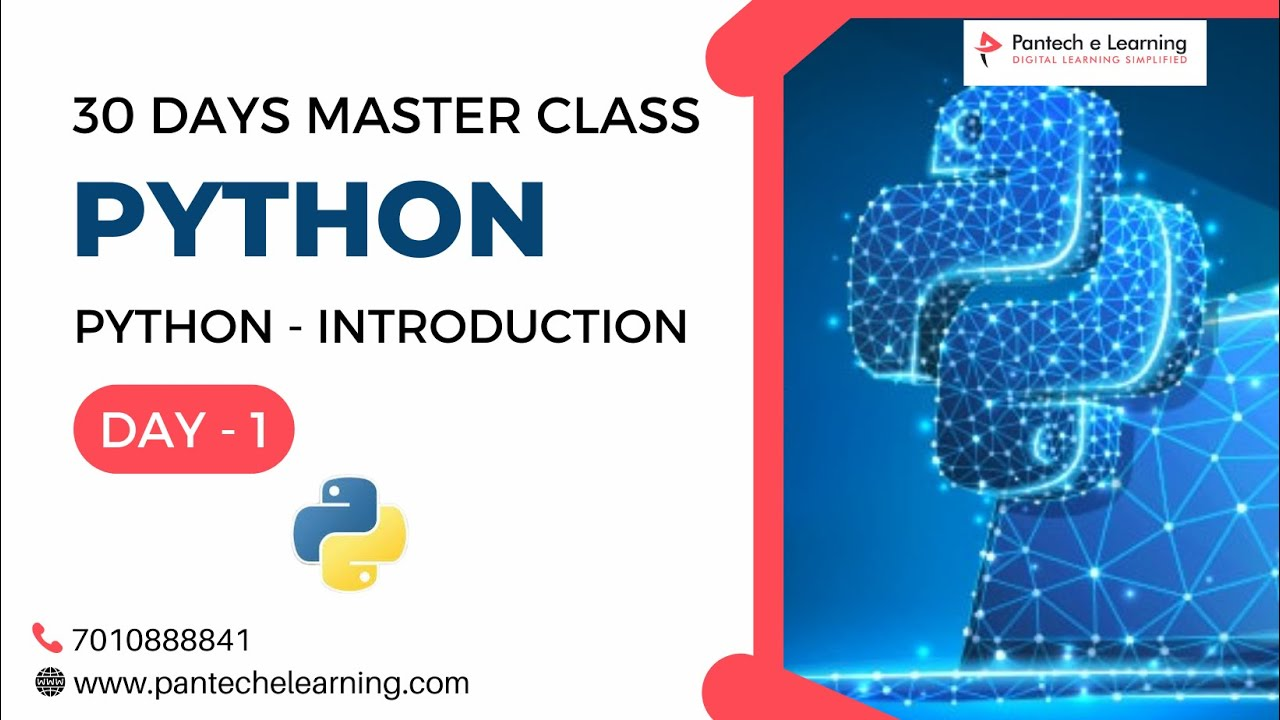# Day 1 Introduction to Python| Python Master ClassDay 1 Introduction to Python| Python Master Class

1 Month Internship on Python Programming
Coupon Code: PYMC
Attn Link: 6.30 PM

Agenda
Day-1 Introduction to Python
Day-2 Python – Tools|Syntaxes & Data Structures
Day-3 Python – Dictionaries,Date and Time
Day-4 Python – MySql
Day-5 Pandas library – Introduction
Day-6 Pandas library – Data Structures
Day-7 Numpy library – Introduction
Day-8 Numpy library – Array Manipulations, Mathematical Functions
Day-9 Matplotlib , Histogram Using Matplotlib | I/O With Numpy
Day-10 Matplotlib Library - Introduction , Pyplot API | Types Of Plots
Day-11 Seaborn Library
Day-12 SKLearn Library
Day-13 Python – Date and Time , Data Wrangling.
Day-14 Python – Data Cleansing
Day-15 Python – Word Tokenization , Stemming and Lammetization.
Day-16 Python – Data Visualization
Day-17 Python – Statistical Analysis
Day-18 Python – Types Of Distribution
Day-19 Python – Correlation ,Chi-Square Test, Linear Regression
Day-20 Keras library – Introduction.
Day-21 Stock Price Prediction With Machine Learning-LSTM RNN
Day-22 People’s behaviour in chat message using NLP-NLP
Day-23 Gaming In Python
Day-24 Chatbot creation in Python-NLP
Day-25 Electricity Price Prediction Using ML-Random Forest Regressor
Day-26 Diamond Price Prediction Using Python-Linear Regression
Day-27 Book Recommendation System-KNN
Day-28 Image Classification Using CNN-CNN
Day-29 Titanic Survival Analysis Using ML-Logistic Regression
Day-30 Blockchain in Python